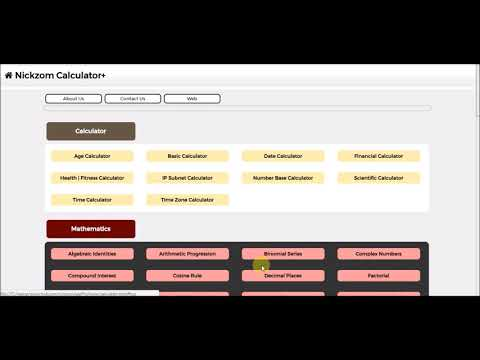# Nickzom Calculator+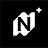10+Everyone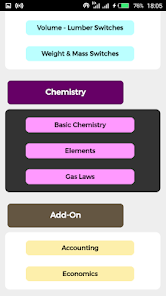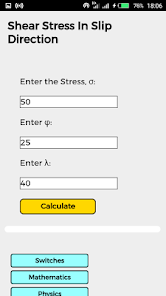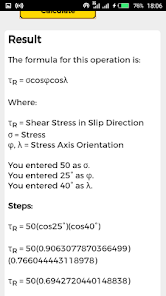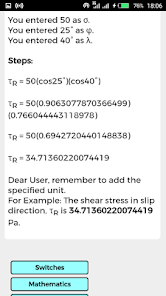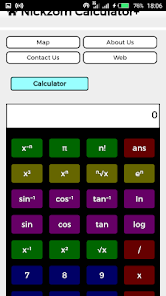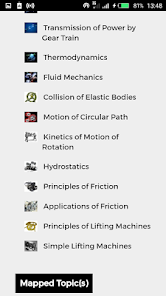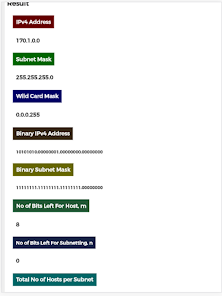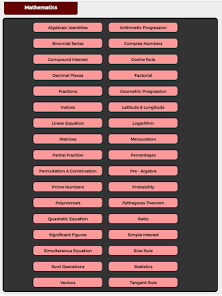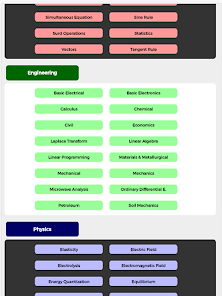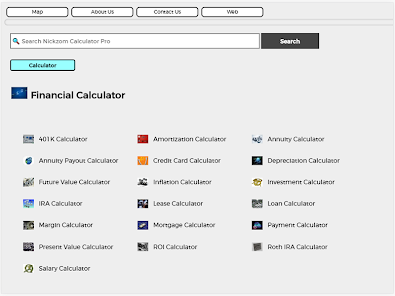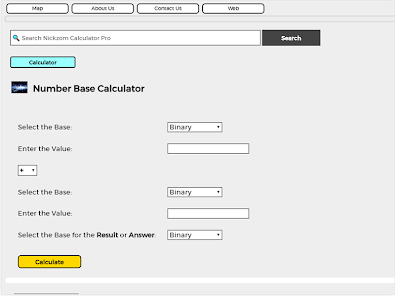Nickzom Calculator+ is an app that performs simple and advanced calculations on Mathematics, Physics, Engineering, Chemistry and Switches or Unit Conversions and lays out the solution(s) and steps for quick and easy understanding.

This app also has Age, Basic, BMI, Date, Financial, Time, Time Zone, IP Subnet, Number Base and Scientific calculator and 30+ Unit Converters.

In Engineering, Nickzom Calculator+ covers:

Economics
Basic Electrical & Electronic
Calculus
Chemical
Civil
Laplace Transform
Linear Algebra
Linear Programming
Materials & Metallurgical
Mechanical
Microwave analysis
Ordinary Differential Equation
Soil Mechanics

There are so many other sub topics in this major topics. For Example in Linear Algebra, Calculator+ can calculate Matrix Arithmetic, Inverse of a Matrix, Determinant of a Matrix, Transpose of a matrix, Adjoint of a matrix, Minors and Cofactors of a matrix. It can also solve simultaneous equation using matrix method. Not only that in matrix arithmetic, one can perform any of this operation: Addition and Subtraction of a matrix, Multiplication of matrices and a matrix by a scalar, Division of a matrix.

In Mathematics, Nickzom Calculator+ covers with there are many sub topics under too:

Algebraic Identities
Arithmetic Progression
Binomial Series
Complex Numbers
Compound Interest
Cosine Rule
Decimal Places
Factorial
Fractions
Geometric Progression
Indices
Latitude and Longitude
Linear Equation
Logarithm
Matrices
Mensuration
Partial Fraction
Percentages
Permutation and Combination
Pre-Algebra
Prime Numbers
Probability
Polynomials
Pythagoras Theorem
Ratio
Significant Figures
Simple Interest
Simultaneous Equation
Sine Rule
Surd Operations
Statistics
Vectors
Tangent Rule

For Example: In Statistics, Nickzom Calculator+ can perform operations with discrete data with and without frequencies and continuous data. Measures of Central Tendency, Measures of Dispersion and other measures such as Skewness, Kurtosis, Moment, Correlation and Regression.

In Physics, Nickzom Calculator+ covers:

Elasticity
Electric Field
Electrolysis
Electromagnetic
Energy Quantization
Equilibrium Of Forces
Gas Laws
Gravitational Field
Heat Energy
Machines
Magnetic Field
Motion
Pressure
Projectile
Resistivity
Simple A.C. Circuit
Vectors
Waves
Wave-Particle Behaviour
Work, Energy and Power

For Example: In Motion, Nickzom Calculator+ can handle finding all the parameters of the first four equations of motion and other parameters such as reaction, time, frequency, angular velocity, angular displacement etc.

In Switches or Unit Conversions, Nickzom Calculator+ covers:

Angle Switches
Area Switches
Base Switches
Coordinates Switches
Data Switches
Data Transfer Rate Switches
Electricity Switches
Energy Switches
Engineering Switches
Fluid Switches
Force Switches
Fraction Switches
Frequency Switches
Fuel Economy Switches
Heat Switches
Length Switches
Light Switches
Magnetism Switches
Prefix Switches
Pressure Switches
Power Switches
Sound Switches
Speed Switches
Temperature Switches
Time Switches
Typography Switches
Volume Switches
Volume - Lumber Switches
Weight & Mass Switches

For Example: In Magnetism Switches, Nickzom Calculator+ can handle magnetomotive force switches, magnetic field strength switches, magnetic flux switches, magnetic flux density switches.

In Chemistry, Nickzom Calculator+ covers:

Basic Chemistry (50+ chemistry solutions)
Such as: Kinetic Theory of Gases, Ideal Gas Equation, Graham's Law of Diffusion, Dilution Equation, Solution Purity, Empirical Formula, Yield, Electric Force and Current, Electrolysis, De Broglie's Law, Density, Number of particles in a substance, Linear Momentum, Percentage Composition of an Element, Mass, Molar Mass, Number of Moles and so much more.
Element Information such as group number, atomic weight and number, boiling and melting point, symbol and specific heat.
Gas Laws

Nickzom Calculator+ requires no internet connection.
Updated on
Feb 10, 2022

## Data safety